﻿ Analytical modeling (AMWELLS) > Pit dewatering > Dewatering by in-pit sumps

# Dewatering by in-pit sumps

Navigation:  Analytical modeling (AMWELLS) > Pit dewatering >

# Dewatering by in-pit sumps

ANSPIT calculates groundwater inflows to an open pit based on a selected conceptual scheme, the pit layout, specified time and target drawdowns. It is assumed that dewatering is achieved by in-pit sumps. For the analytical schemes of unconfined aquifer and linear source in confined aquifer, the algorithm takes into account the excavation depth of the pit.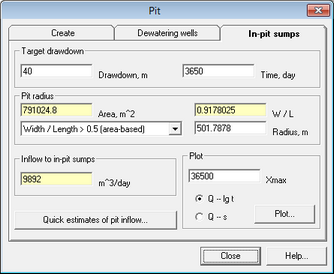"In-pit sumps" tab

 "Target drawdown" frame Defines target drawdown and the time, by which the target has to be achieved (based on excavation schedule) "Pit radius" frame Determines effective radius of the pit. It can be entered manually or ANSPIT can calculate this value based on entered parameters of pit layout List You can specify effective radius (ro) using one of the following five options: 1. Manually – by entering value in the text box "Radius". 2. Width / Length > 0.5 (area-based) – radius for non-elongated layouts is calculated using area of the pit (F) by the formula:3. Width / Length > 0.5 (perimeter-based) – radius for non-elongated layouts is calculated using perimeter of the pit contour (P) by the formula: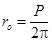4. Width / Length <= 0.5 – radius for elongated layouts is calculated by the formula: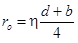d – pit length, m; b – pit width, m; η – it is defined by a polynomial: for b / d <= 0.5: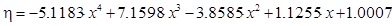5. Complex layout – radius is calculated using the formula: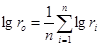n – number of polygon segments; ri – distance from pit center to vertex of polygon "Area" or "Perimeter" text box Information field. Shows the pit area (or perimeter, if the perimeter-based formula is chosen). The value is used for determination of the effective radius for non-elongated pit "Width / length" ratio text box Information field. Shows the ratio between width and length of the pit. The value is used for determination of the effective radius for elongated pit "Inflow to in-pit sumps" frame Field shows the calculated pit inflow (m3/d) for in-pit sumps with de-activated abstraction wells. CTRL-LMB – opens the conversion table for time units. If a pit inflow value is entered in this field, the pit drawdown will be calculated and displayed in the frame "Target drawdown" "Plot" frame Fields and options for two types of plots: 1) hydrograph of pit inflow; 2) pit inflow versus drawdown "Xmax" text box Maximum bound of X-axis. Minimum bound is assigned equal to the values of time or drawdown entered in frame "Target drawdown" "Q - lg t" option Plots pit inflow versus logarithmic time. Time values range from the value assigned in text box "Time" of frame "Target drawdown" to the value specified in the text box "Xmax" "Q - s" option Plots pit inflow versus drawdown. Time values range from the value assigned in text box "Drawdown" of frame "Target drawdown" to the value specified in the text box "Xmax" "Plot" button Creates plots "Quick estimates of pit inflow" button Opens the window dialog "Pit inflow". Calculates inflow rates to in-pit sumps using referenced analytical solutions